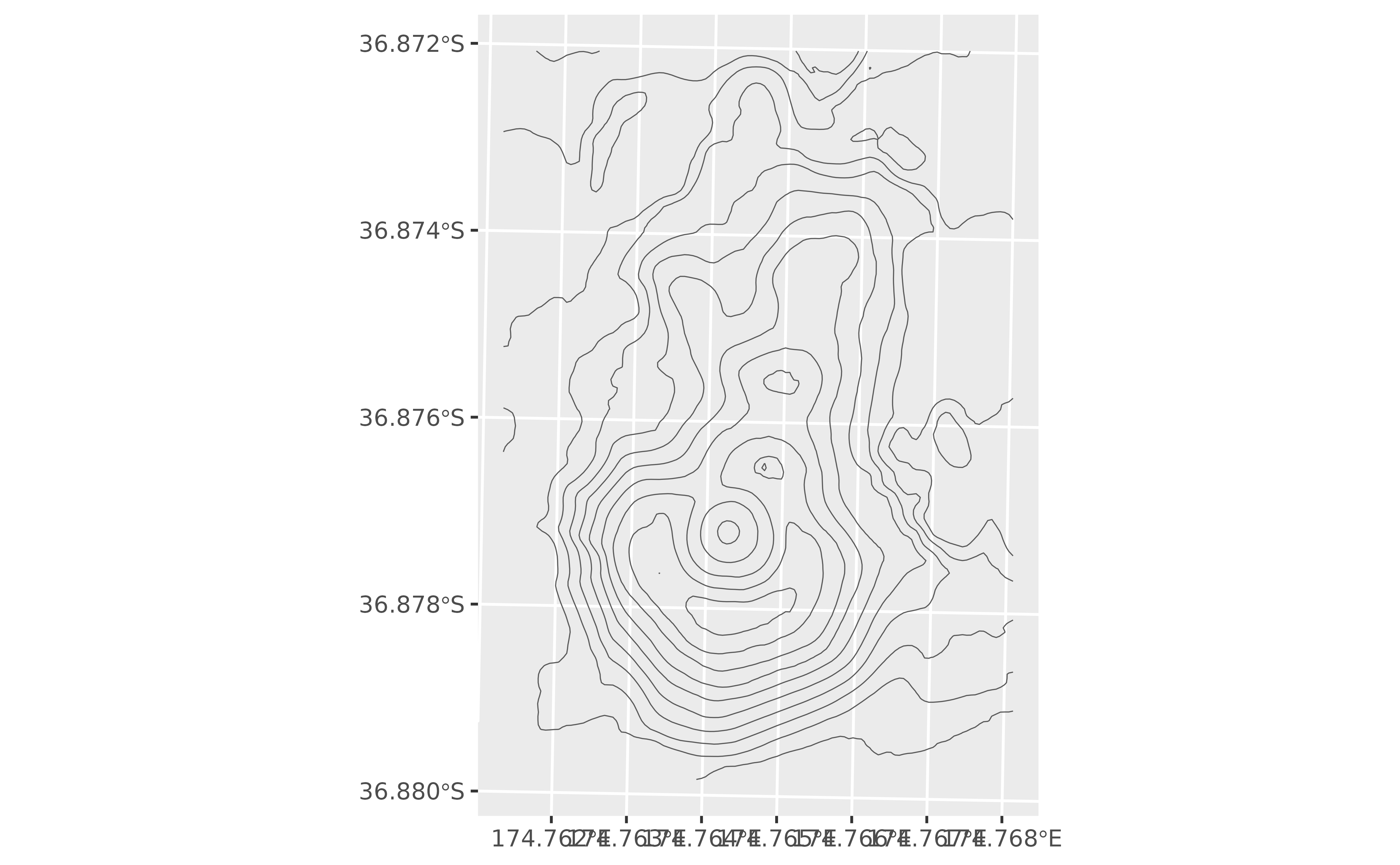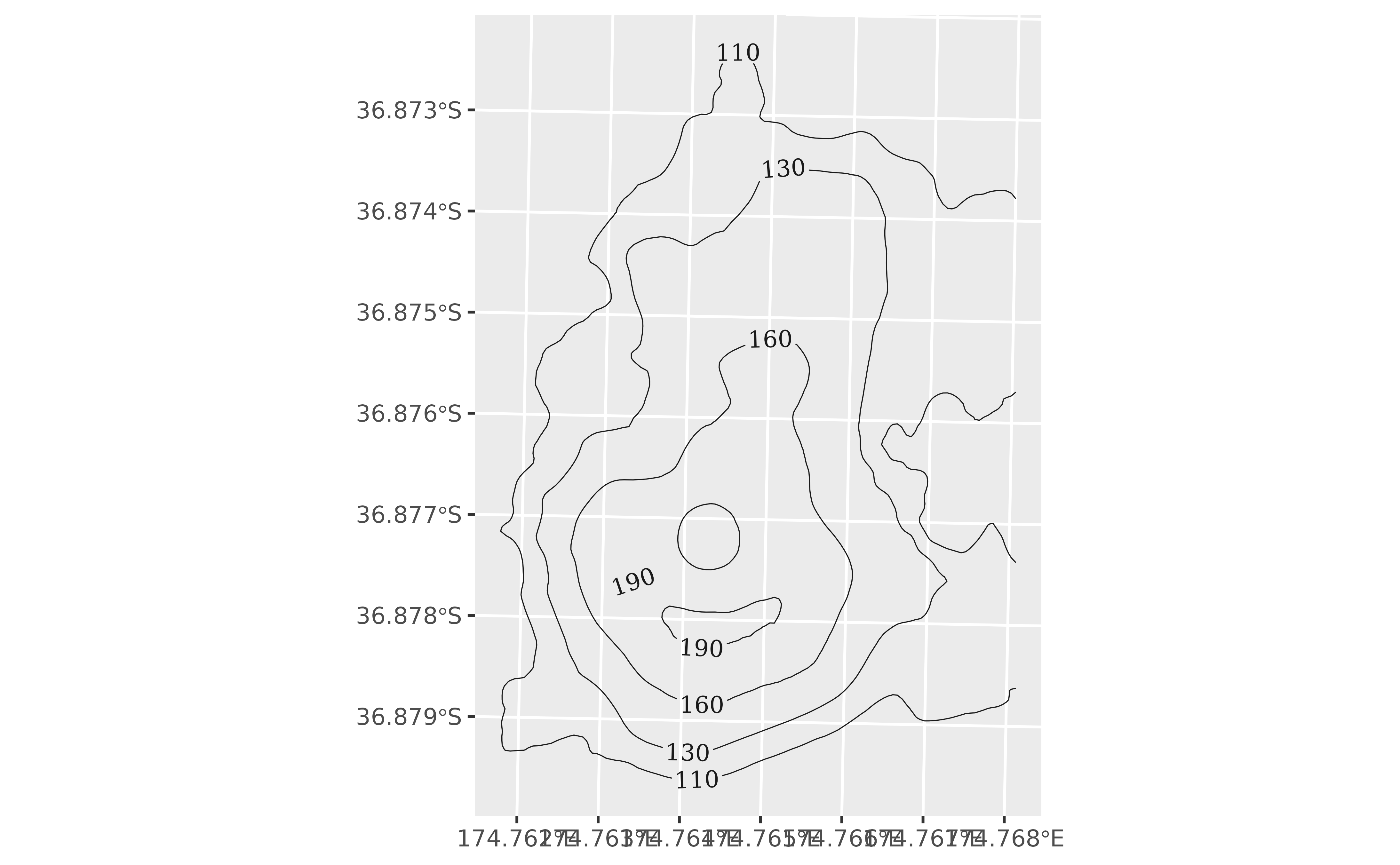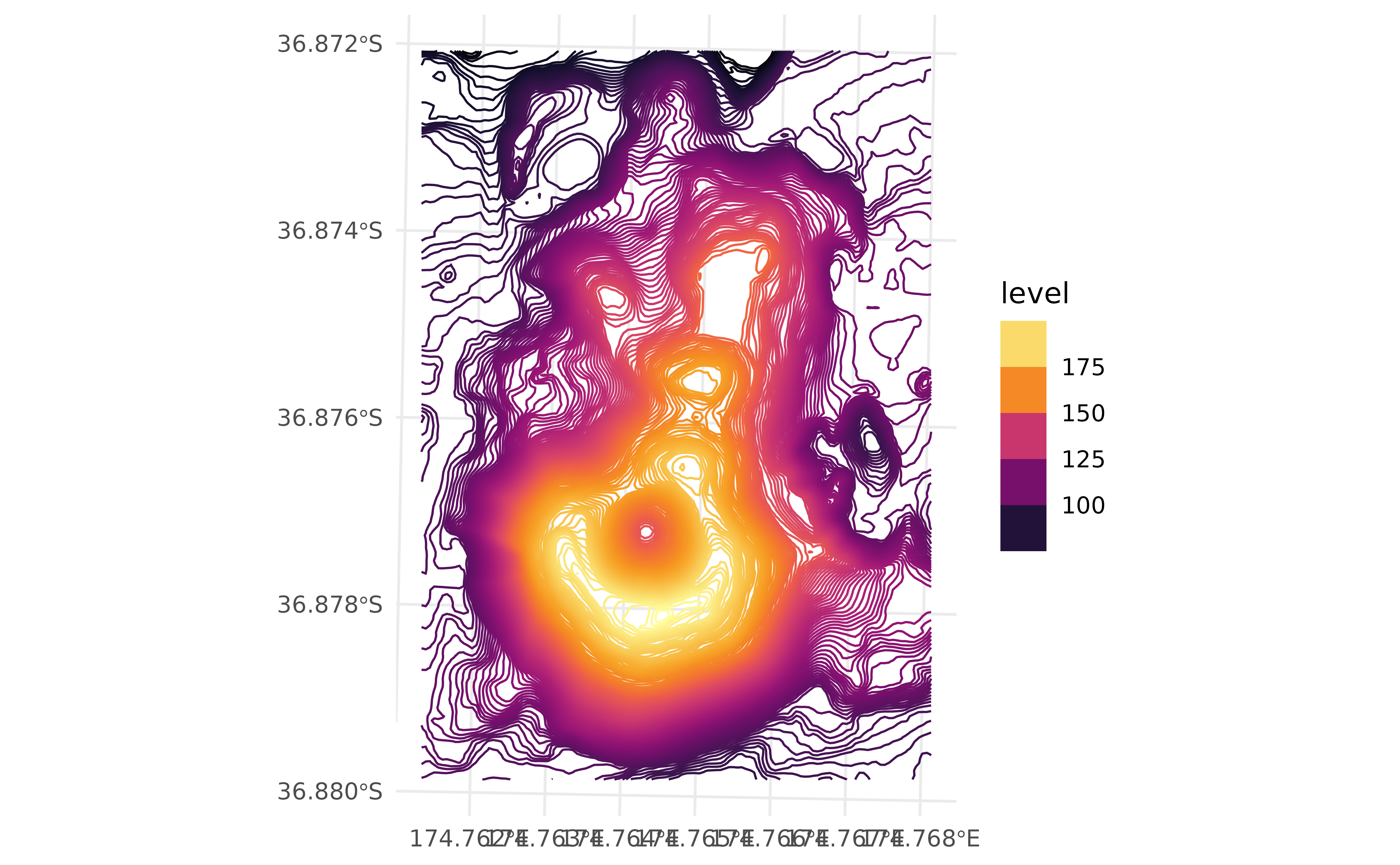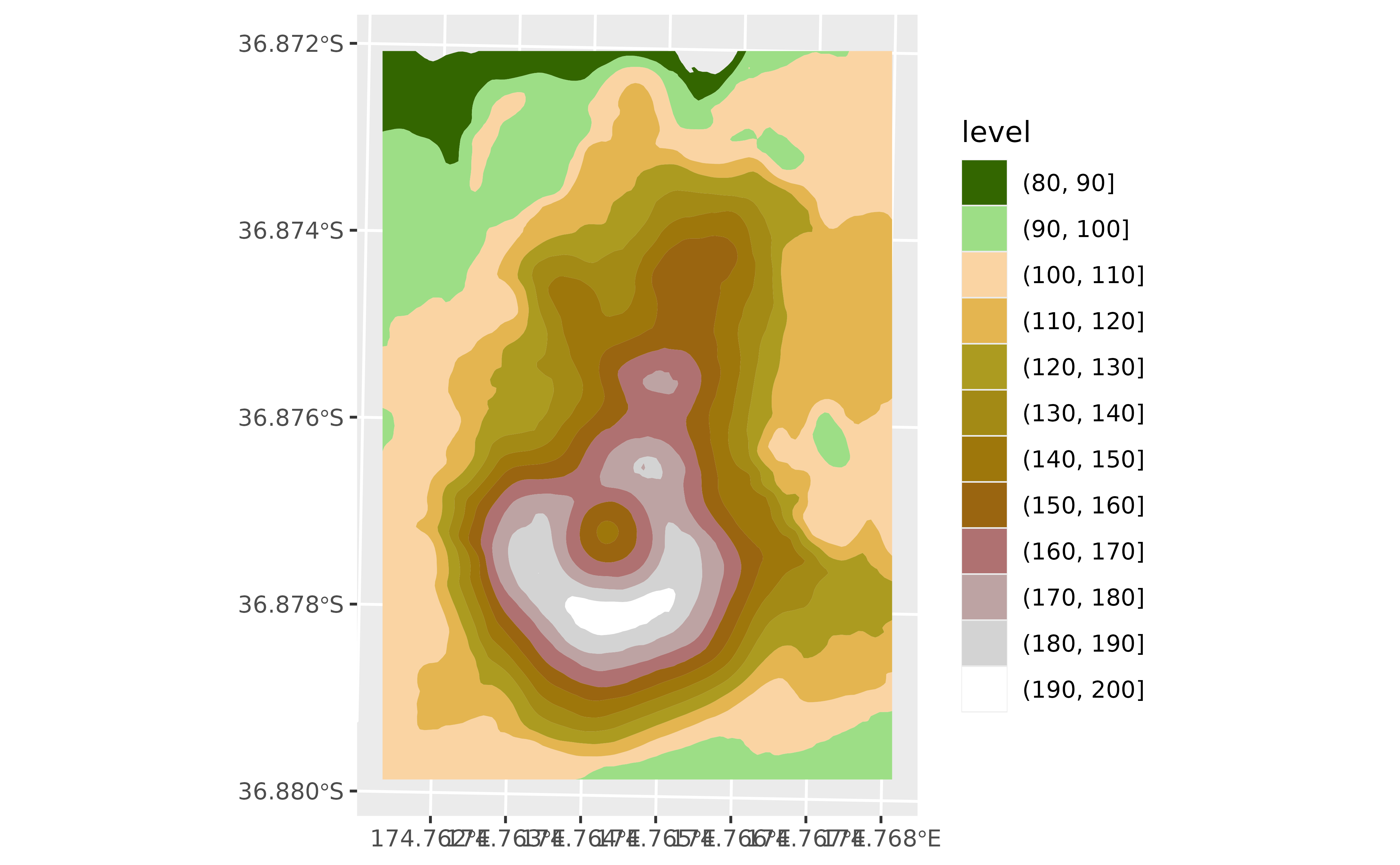These geoms create contours of SpatRaster objects. To specify a valid surface, you should specify the layer on aes(z = layer_name), otherwise all the layers would be consider for creating contours. See also Facets section.

The underlying implementation is based on ggplot2::geom_contour().

## Usage

geom_spatraster_contour(
mapping = NULL,
data,
...,
maxcell = 5e+05,
bins = NULL,
binwidth = NULL,
breaks = NULL,
na.rm = TRUE,
show.legend = NA,
inherit.aes = TRUE
)

geom_spatraster_contour_filled(
mapping = NULL,
data,
...,
maxcell = 5e+05,
bins = NULL,
binwidth = NULL,
breaks = NULL,
na.rm = TRUE,
show.legend = NA,
inherit.aes = TRUE
)

## Arguments

mapping

Set of aesthetic mappings created by ggplot2::aes() or ggplot2::aes_(). See Aesthetics specially in the use of fill aesthetic.

data

A SpatRaster object.

...

Other arguments passed on to layer(). These are often aesthetics, used to set an aesthetic to a fixed value, like colour = "red" or size = 3. They may also be parameters to the paired geom/stat.

maxcell

positive integer. Maximum number of cells to use for the plot.

bins

Number of contour bins. Overridden by binwidth.

binwidth

The width of the contour bins. Overridden by breaks.

breaks

One of:

• Numeric vector to set the contour breaks

• A function that takes the range of the data and binwidth as input and returns breaks as output. A function can be created from a formula (e.g. ~ fullseq(.x, .y)).

Overrides binwidth and bins. By default, this is a vector of length ten with pretty() breaks.

na.rm

If TRUE, the default, missing values are silently removed. If FALSE, missing values are removed with a warning.

show.legend

logical. Should this layer be included in the legends? NA, the default, includes if any aesthetics are mapped. FALSE never includes, and TRUE always includes. It can also be a named logical vector to finely select the aesthetics to display.

inherit.aes

If FALSE, overrides the default aesthetics, rather than combining with them.

A ggplot2 layer

## terra equivalent

terra::contour()

## Aesthetics

geom_spatraster_contour() understands the following aesthetics:

• alpha

• colour

• group

• linetype

• linewidth

• weight

Additionally, geom_spatraster_contour_filled() understands also the following aesthetics, as well as the ones listed above:

• fill

• subgroup

Check ggplot2::geom_contour() for more info.

## Computed variables

This geom computes internally some variables that are available for use as aesthetics, using (for example) aes(color = after_stat(<computed>)) (see ggplot2::after_stat()).

level

Height of contour. For contour lines, this is numeric vector that represents bin boundaries. For contour bands, this is an ordered factor that represents bin ranges.

nlevel

Height of contour, scaled to maximum of 1.

lyr

Name of the layer.

level_low, level_high, level_mid

(contour bands only) Lower and upper bin boundaries for each band, as well the mid point between the boundaries.

## Coords

When the SpatRaster does not present a crs (i.e., terra::crs(rast) == "") the geom does not make any assumption on the scales.

On SpatRaster that have a crs, the geom uses ggplot2::coord_sf() to adjust the scales. That means that also the SpatRaster may be reprojected.

## Facets

You can use  facet_wrap(~lyr) for creating a faceted plot by each layer of the SpatRaster object. See ggplot2::facet_wrap() for details.

ggplot2::geom_contour()

Other ggplot2 utils: autoplot.Spat, fortify.Spat, geom_spatraster_rgb(), geom_spatraster(), ggspatvector, stat_spat_coordinates()

## Examples

# \donttest{

library(terra)

# Raster
f <- system.file("extdata/volcano2.tif", package = "tidyterra")
r <- rast(f)

library(ggplot2)

ggplot() +
geom_spatraster_contour(data = r)ggplot() +
geom_spatraster_contour(
data = r, aes(color = after_stat(level)),
binwidth = 1,
linewidth = 0.4
) +
colours = hcl.colors(20, "Inferno"),
guide = guide_coloursteps()
) +
theme_minimal()# Filled with breaks
ggplot() +
geom_spatraster_contour_filled(data = r, breaks = seq(80, 200, 10)) +
scale_fill_hypso_d()# Both lines and contours
ggplot() +
geom_spatraster_contour_filled(
data = r, breaks = seq(80, 200, 10),
alpha = .7
) +
geom_spatraster_contour(
data = r, breaks = seq(80, 200, 2.5),
color = "grey30",
linewidth = 0.1
) +
scale_fill_hypso_d()# }